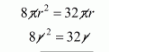# If four times the sum of the areas of two circular faces`
Question:

If four times the sum of the areas of two circular faces of a cylinder of height 8 cm is equal to twice the curve surface area, then diameter of the cylinder is

(a) 4 cm

(b) 8 cm

(c) 2 cm

(d) 6 cm

Solution:

Let r be the radius of cylinder.

Area of circular base of cylinderThe height of cylinder h = 8 cm

The C.S.A. of cylinderClearly,

$4 \times\left(\pi r^{2}+\pi r^{2}\right)=2 \times(\pi r)$The diameter of cylinderHence, the correct answer is choice (b).